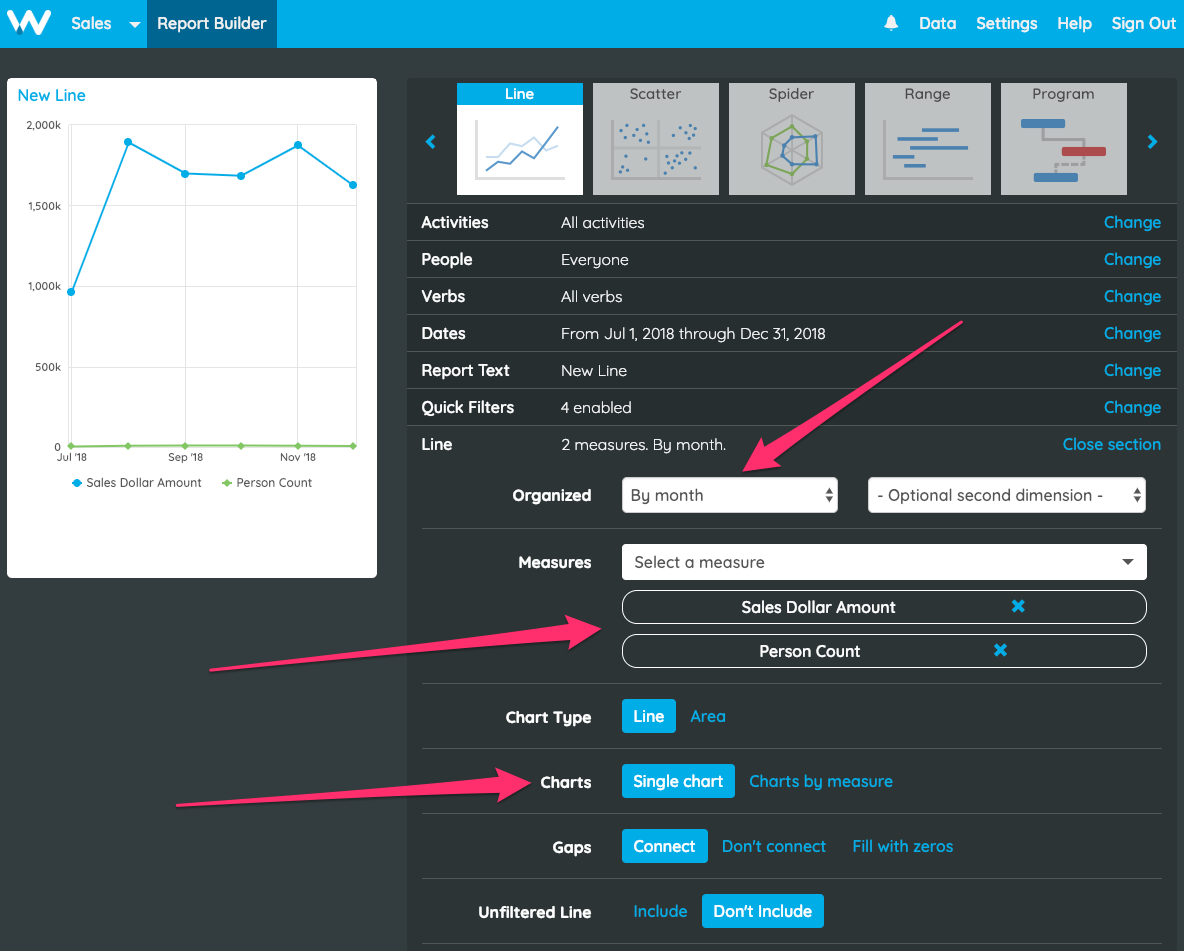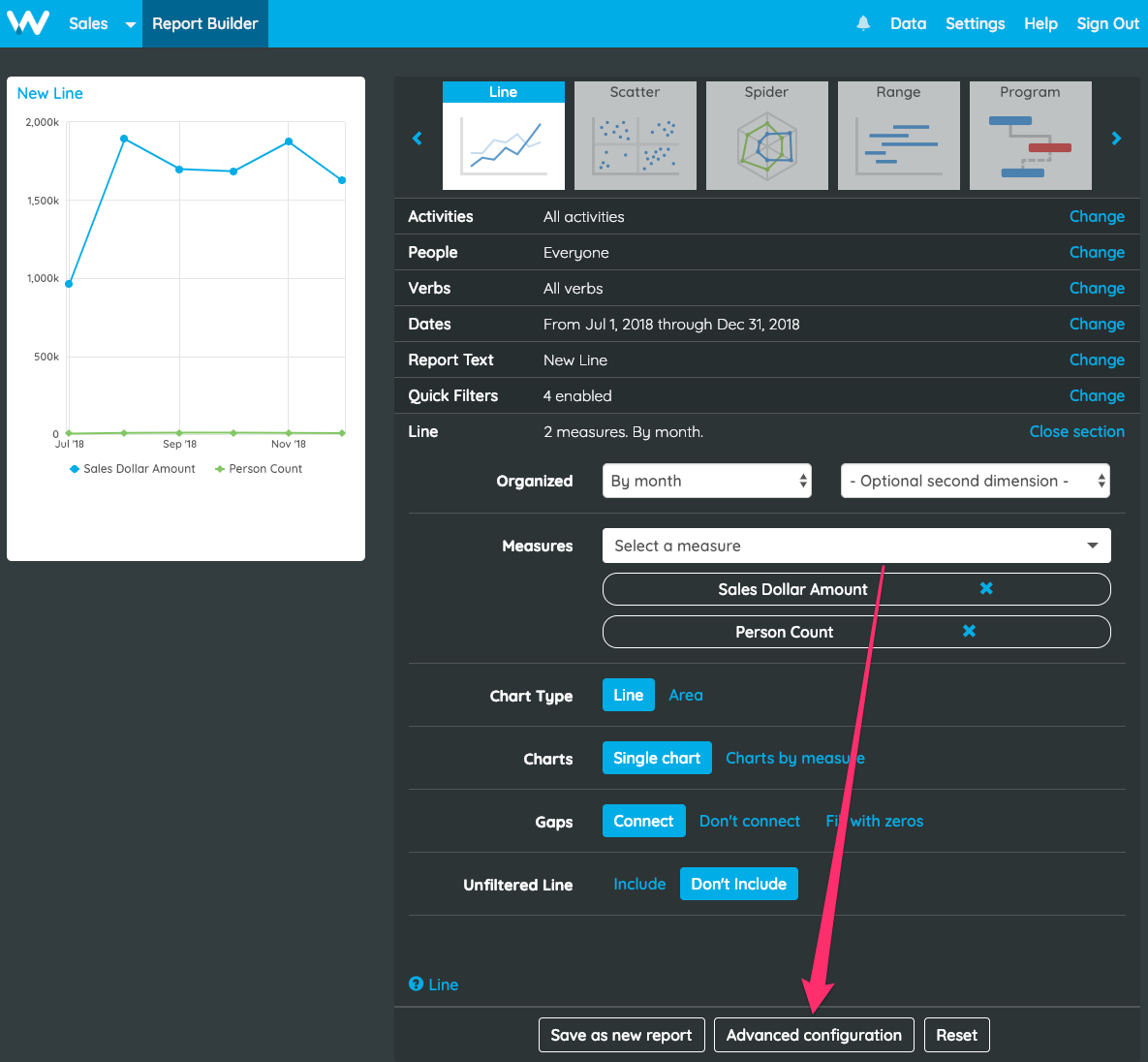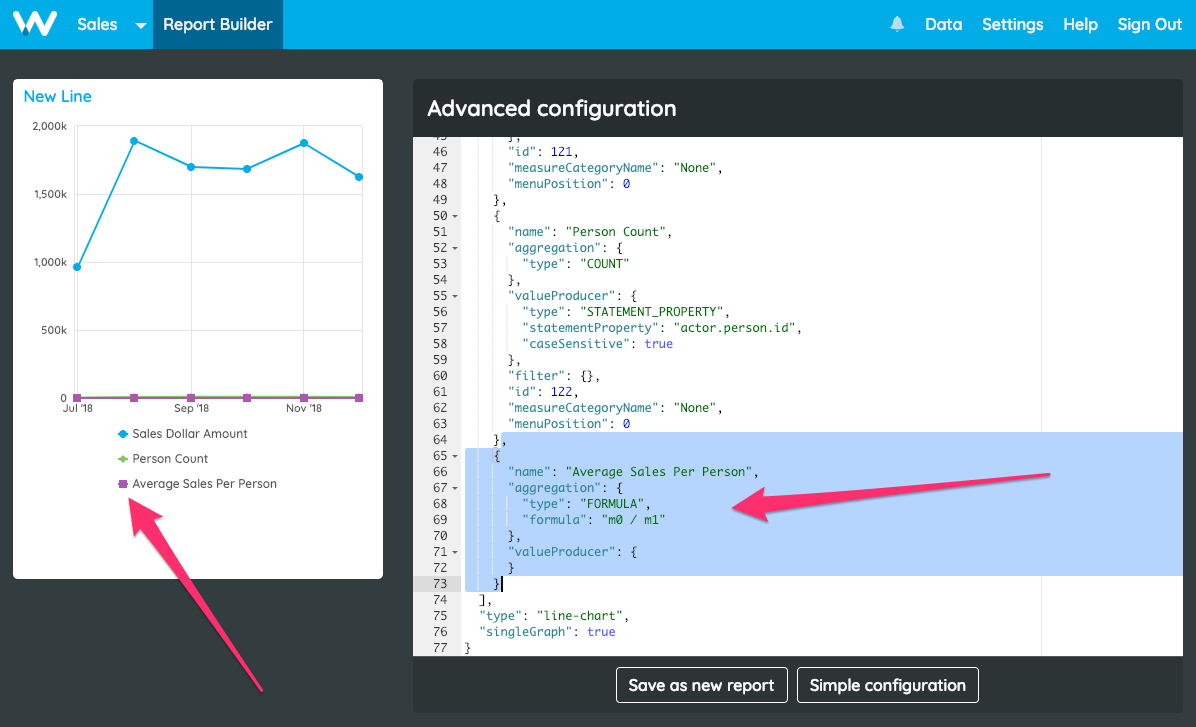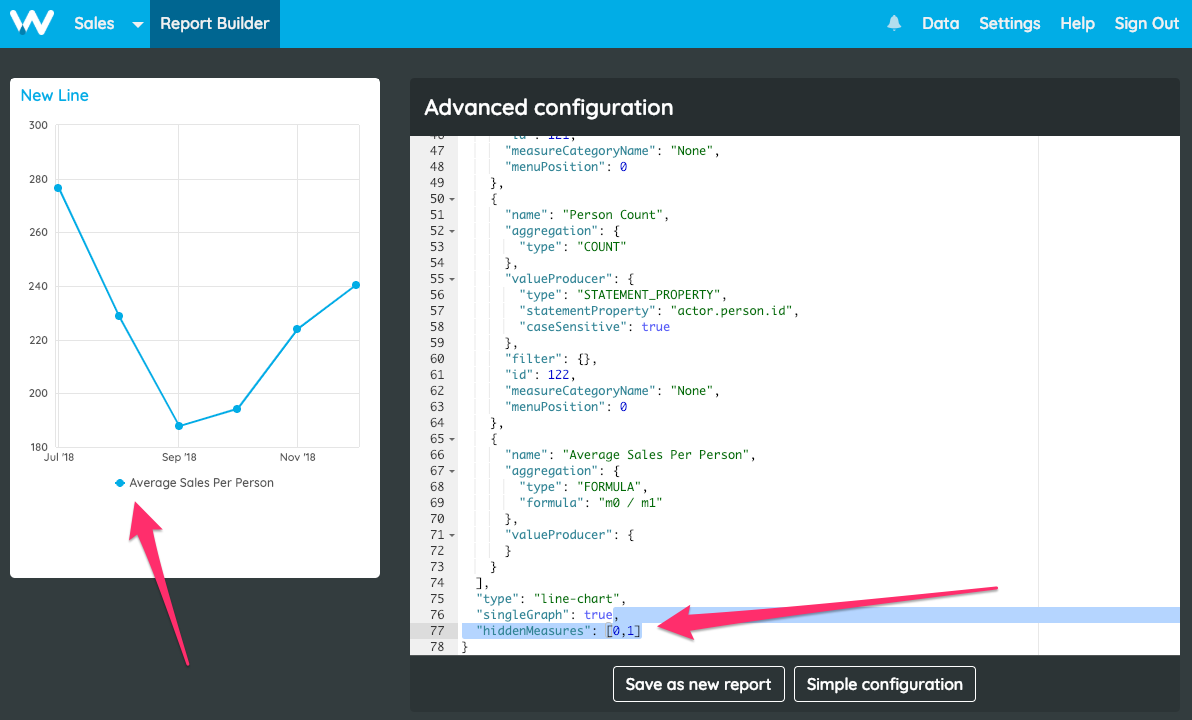# How do I perform calculations with Formula Measures?

Watershed measures enable you to perform aggregations based on your data. Taking a filtered data set, you can use measures to get the sum, average, min, max, first, last, count and distinct count of any xAPI statement property.

When you want to perform more complex calculations, the formula measure can be used to combine multiple measures to perform complex calculations. You can use it to calculate thing like ROI, average monthly total sales per person or completions as a percentage of enrollments. The details of available calculations are outlined in the measure editor reference guide. This walkthrough guide takes you through the process of implementing a simple division to calculate average total sales per person in a line chart organized by month. This is different from average sales, as that would show you the average per deal, rather than per person.

Who can use this feature?
User Types
Admin users can create and edit measures.
Pricing
Available on paid plans (AnalystCLO, and Enterprise).
Expertise
Only experts can use this feature.

# Step 1: Create the Report

Let’s assume you’ve already got data about sales in your account and have person count and total sales measures already created. If you don’t have those measures in your account, use someother measures that it might be interesting to perform a calculation with.

Your first step is to create a line chart organized by month that uses those two measures.Once you’re done, jump into advanced configuration for step 2.# Step 2: Add the Formula measure

Now we can add the formula measure to calculate total sales divided by person count, i.e. average total sales per person. Don’t forget to include the comma before adding the measure below as the third and final measure.

```{
"name": "Average Sales Per Person",
"aggregation": {
"type": "FORMULA",
"formula": "m0 / m1"
},
"valueProducer": {
}
}```

This formula calculates the first measure divided by the second measure. Measures are denoted in the formula by the letter m followed by the measure index. So the first measure is m0, the second measure is m1, the third measure is m2 etc.

Please note: Formula Measures are the result of calculations between measures on a report. The calculations can only be performed between measures whose configurations are in the same report as the Formula Measure.# Step 3: Hide the other measures

We’re now calculating average sales per person, but the figure is dwarfed by the total sales measure and therefore not displayed nicely on the chart. We need to hide the other two measures. This is done using the hiddenMeasures property, which you will need to add to the report configuration object if it’s not already there. Set the value of this property to [0,1] to tell Watershed that you want to hide the 0 and 1 indexed measures:

`"hiddenMeasures": [0,1]`That’s it! Save your report and you’re done!